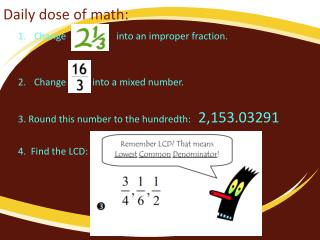DownloadDownload PresentationDaily dose of math:

# Daily dose of math:

Télécharger la présentation## Daily dose of math:

- - - - - - - - - - - - - - - - - - - - - - - - - - - E N D - - - - - - - - - - - - - - - - - - - - - - - - - - -
##### Presentation Transcript

1. Daily dose of math: Change into an improper fraction. Change into a mixed number. 3. Round this number to the hundredth: 2,153.03291 4. Find the LCD:

2. Adding & Subtracting Fractions * My goal is... to successfully add and subtract similar and dissimilar fractions without a calculator. What is the rule when adding or subtracting fractions? -Fractions must first have the same denominator before they can be added together or subtracted from one another. Fractions with the same denominator are called similar fractions.

3. Adding & Subtracting Fractions * My goal is... to successfully add and subtract similar and dissimilar fractions without a calculator. What is the rule when adding or subtracting fractions? -Fractions must first have the same denominator before they can be added together or subtracted from one another. Fractions with the same denominator are called similar fractions.

4. Adding & Subtracting Fractions * My goal is... to successfully add and subtract similar and dissimilar fractions without a calculator. What is the rule when adding or subtracting fractions? -Fractions must first have the same denominator before they can be added together or subtracted from one another. Fractions with the same denominator are called similar fractions.

5. Adding and Subtracting (with different denominators) • For fractions with different denominators, you must find EQUIVALENT fractions with the SAME denominator before you can add or subtract. • Notes CONTINUED: • Once you find the LCD (lowest common denominator), you must find the adjust the numerators. • Then line the fractions up vertically (first one on top of the second one). • Finally, add or subtract the numerators, and keep the denominator the same. • Be sure to always SIMPLIFY an answer that is a fraction. If improper, turn it into a mixed number.

6. WORD PROBLEMS Jalil hiked miles on Saturday and miles on Sunday. How much farther did he hike on Saturday than Sunday?

7. Classwork: Find the sum or difference. Remember to find the least common denominator.

8. Daily Dose: • What fraction problem is shown in the picture below? Hint- Use mixed numbers in your problem. Then solve. Write down the problem and determine what the answer will be. • Add: • Subtract:

9. Adding and Subtracting Mixed Numbers! * My goal is... to successfully add and subtract MIXED NUMBERS without a calculator.